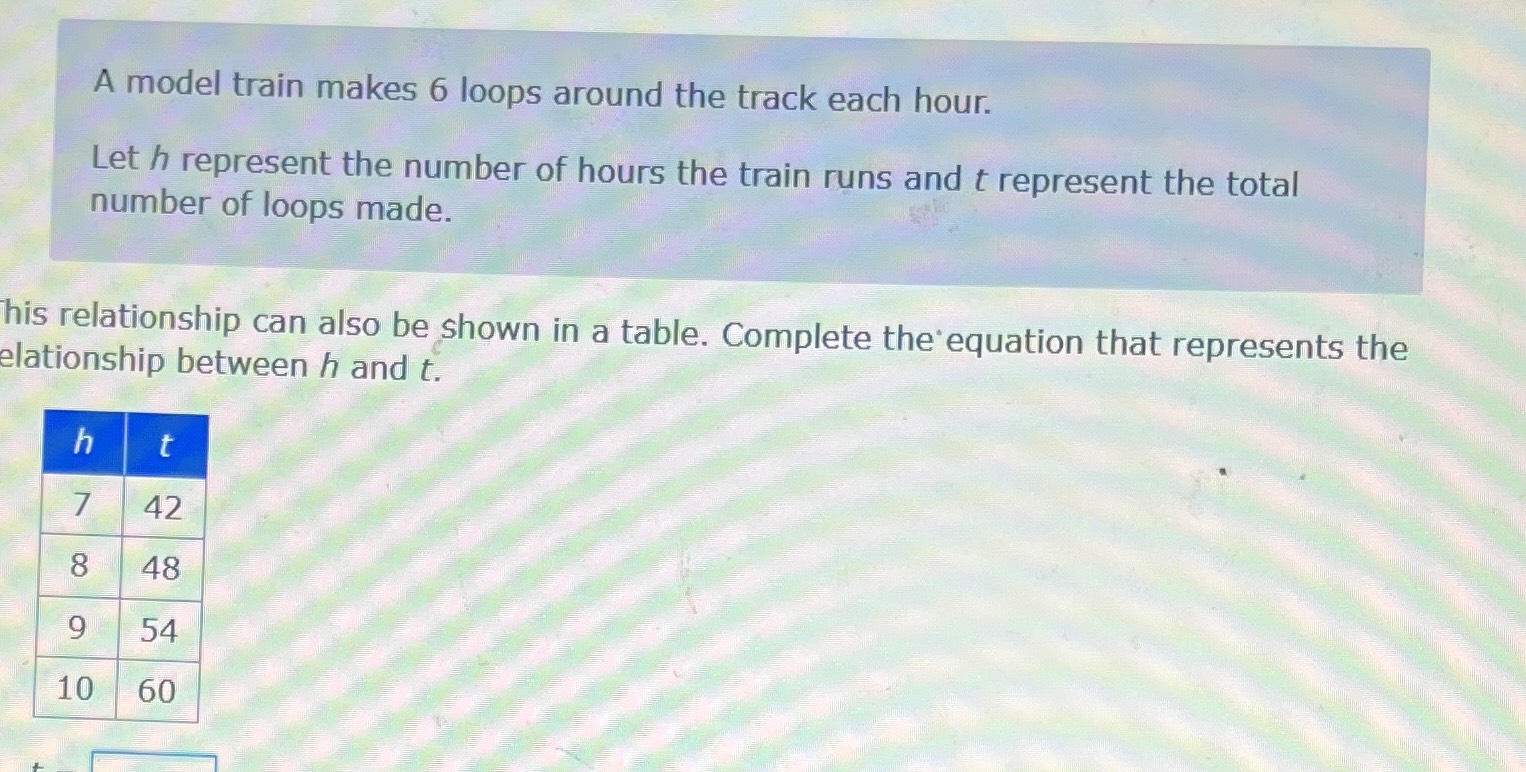### ¿Todavía tienes preguntas de matemáticas?

Pregunte a nuestros tutores expertos
Algebra
PreguntaA model train makes 6 loops around the track each hour.

Let $$h$$ represent the number of hours the train runs and $$t$$ represent the total number of loops made.

This relationship can also be shown in a table. Complete the equation that represents the relationship between $$h$$ and $$t$$ .